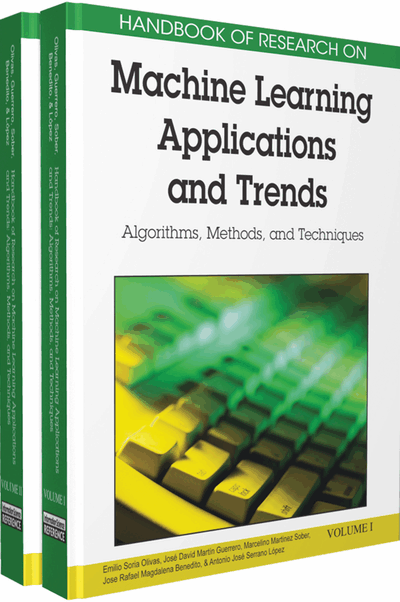# Principal Graphs and Manifolds

Alexander N. Gorban (University of Leicester, UK) and Andrei Y. Zinovyev (Institut Curie, France)
DOI: 10.4018/978-1-60566-766-9.ch002
Available
\$37.50
No Current Special Offers

## Abstract

In many physical, statistical, biological and other investigations it is desirable to approximate a system of points by objects of lower dimension and/or complexity. For this purpose, Karl Pearson invented principal component analysis in 1901 and found ‘lines and planes of closest fit to system of points’. The famous k-means algorithm solves the approximation problem too, but by finite sets instead of lines and planes. This chapter gives a brief practical introduction into the methods of construction of general principal objects (i.e., objects embedded in the ‘middle’ of the multidimensional data set). As a basis, the unifying framework of mean squared distance approximation of finite datasets is selected. Principal graphs and manifolds are constructed as generalisations of principal components and k-means principal points. For this purpose, the family of expectation/maximisation algorithms with nearest generalisations is presented. Construction of principal graphs with controlled complexity is based on the graph grammar approach.
Chapter Preview
Top

## Introduction

In many fields of science one meets with multivariate (multidimensional) distributions of vectors representing some observations. These distributions are often difficult to analyse and make sense of due to the very nature of human brain which is able to visually manipulate only with the objects of dimension no more than three.

This makes actual the problem of approximating the multidimensional vector distributions by objects of lower dimension and/or complexity while retaining the most important information and structures contained in the initial full and complex data point cloud.

The most trivial and coarse approximation is collapsing the whole set of vectors into its mean point. The mean point represents the ‘most typical’ properties of the system, completely forgetting variability of observations.

The notion of the mean point can be generalized for approximating data by more complex types of objects. In 1901 Pearson proposed to approximate multivariate distributions by lines and planes (Pearson, 1901). In this way the Principal Component Analysis (PCA) was invented, nowadays a basic statistical tool. Principal lines and planes go through the ‘middle’ of multivariate data distribution and correspond to the first few modes of the multivariate Gaussian distribution approximating the data.

Starting from 1950s (Steinhaus, 1956; Lloyd, 1957; and MacQueen, 1967), it was proposed to approximate the complex multidimensional dataset by several ‘mean’ points. Thus k-means algorithm was suggested and nowadays it is one of the most used clustering methods in machine learning (see a review presented by Xu & Wunsch, 2008).

Both these directions (PCA and K-Means) were further developed during last decades following two major directions: 1) linear manifolds were generalised for non-linear ones (in simple words, initial lines and planes were bended and twisted), and 2) some links between the ‘mean’ points were introduced. This led to appearance of several large families of new statistical methods; the most famous from them are Principal Curves, Principal Manifolds and Self-Organising Maps (SOM). It was quickly realized that the objects that are constructed by these methods are tightly connected theoretically. This observation allows now to develop a common framework called “Construction of Principal Objects”. The geometrical nature of these objects can be very different but all of them serve as data approximators of controllable complexity. It allows using them in the tasks of dimension and complexity reduction. In Machine Learning this direction is connected with terms ‘Unsupervised Learning’ and ‘Manifold Learning.’

In this chapter we will overview the major directions in the field of principal objects construction. We will formulate the problem and the classical approaches such as PCA and k-means in unifying framework, and show how it is naturally generalised for the Principal Graphs and Manifolds and the most general types of principal objects, Principal Cubic Complexes. We will systematically introduce the most used ideas and algorithms developed in this field.

## Key Terms in this Chapter

Principal Components: Such an orthonormal basis in which the covariance matrix is diagonal.

Principal Graph: A graph embedded in the multidimensional data space, providing the minimal mean squared distance to the dataset combined with deviation from an “ideal” configuration (for example, from pluriharmonic graph) and not exceeding some limits on complexity (in terms of the number of structural elements and the number of graph grammar transformations needed for obtaining the principal graph from some minimal graph).

Principal Manifold: Intuitively, a smooth manifold going through the middle of data cloud; formally, there exist several definitions for the case of data distributions: 1) Hastie and Stuelze’s principal manifolds are self-consistent curves and surfaces; 2) Kegl’s principal curves provide the minimal mean squared error given the limited curve length; 3) Tibshirani’s principal curves maximize the likelihood of the additive noise data model; 4) Gorban and Zinovyev elastic principal manifolds minimize a mean square error functional regularized by addition of energy of manifold stretching and bending; 5) Smola’s regularized principal manifolds minimize some form of a regularized quantization error functional; and some other definitions.

Expectation/Maximisation Algorithm: Generic splitting algorithmic scheme with use of which almost all algorithms for estimating principal objects are constructed; it consists of two basic steps: 1) projection step, at which the data is projected onto the approximator, and 2) maximization step, at which the approximator is optimized given the projections obtained at the previous step.

Self-Consistent Approximation: Approximation of a dataset by a set of vectors such that every point y in the vector set is a conditional mean of all points from dataset that are projected in y.

## Complete Chapter List

Search this Book:
Reset